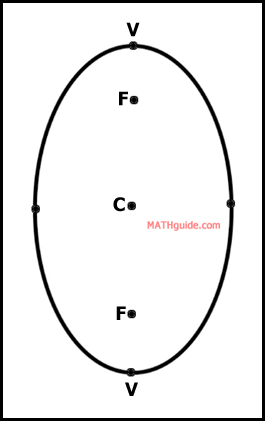Ellipse: Find an Equation Given Vertices & Foci
View the Lesson | MATHguide homepage Updated July 18th, 2023

Status: Read the following problem and respond accordingly. Waiting. . .

Determine the equation of an ellipse with the given information.

The vertices and foci are located at:

V(7,-9), V(7,1),
F(7,-7), F(7,-1).

 (x - + )2 (y - + )2+= 1